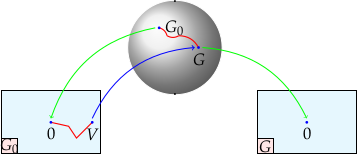# Algorithms

### Rational H2 approximation

Given a matrix-valued function$Latex formula$ whose entries are  assumed to be in the Hardy space 1$Latex formula$ and a positive integer n, the problem is to find a stable2 rational matrix$Latex formula$ of McMillan degree3 at most n which minimizes the$Latex formula$ norm$Latex formula$
It is known  that a global minimum does exist and has exact degree n unless$Latex formula$ is of lower degree. Moreover, if$Latex formula$ has degree n, then the only critical point of the criterion is$Latex formula$ itself . The approach used in RARL2 was first developped for scalar functions  and then in the matrix case .

1. Hardy spaces: the space$Latex formula$ of square integrable functions on the unit disk splits into two orthogonal subspaces: the space$Latex formula$ of$Latex formula$ functions having an analytic continuation in the unit disk (negative Fourier coefficients are zero) and the space$Latex formula$ of$Latex formula$ functions having an analytic continuation outside the unit disk and vanishing at infinity (non-negative Fourier coefficients are zero)

2. stable: poles inside the unit disk

3. McMillan degree: size of the A-matrix in a minimal realization/ number of poles counting multiplicity

### Reduction of the optimization space

We use a special matrix fraction description known has Douglas-Shapiro-Shields factorization, and write a rational matrix of degree n as$Latex formula$
where$Latex formula$ is a lossless matrix4, of same McMillan degree than$Latex formula$, and$Latex formula$ is an anti-stable matrix.

•$Latex formula$ and$Latex formula$: same observable pair$Latex formula$
•$Latex formula$ and$Latex formula$ bring the (left) pole structure
• unique up to a unitary matrix
• right generalization of an irreducible rational fraction

By the Hilbert projection theorem , at a local minimum$Latex formula$ of the criterion,$Latex formula$ has to be the projection$Latex formula$ of$Latex formula$ onto$Latex formula$. The approximation problem reduces to the minimization of a concentrated criterion [4,5]$Latex formula$

over the (quotient) set of lossless matrices of McMillan degree n up to a right unitary matrix.

Advantage: minimization over a bounded set

4. lossless matrix: rationnal matrix analytic outside the unit disk which takes unitary values on the circle.

### State-space formulas

In practice, the concentrated criterion is computed using balanced realizations5 to represent lossless matrix functions. We have the nice following property$Latex formula$

• Write the state-space realization of$Latex formula$ and$Latex formula$.$Latex formula$

where the pair$Latex formula$ is assumed output normal :$Latex formula$
• The Hilbert projection property translates into the classical necessary conditions for optimality$Latex formula$
• The concentrated criterion becomes$Latex formula$

defined over the set of equivalence classes under similarity of balanced output pairs (BOP) .

5. A balanced realization is such that both grammians are equal and diagonal

### Parametrization of the optimization space

The optimization space has a manifold structure . We use an atlas of charts, like for the earth, where each chart provides a local flat (Euclidien) representation which allows for the use of differential tools. In the actual version of RARL2, we use the atlas presented in , called lossless mutual encoding

• A chart is indexed by a lossless matrix/unitary realization$Latex formula$
• A BOP, given by a unitary realization$Latex formula$ can be parametrized in this chart iff the solution$Latex formula$ to$Latex formula$

is positive definite.
• The BOP is represented in the chart by the parameters matrix$Latex formula$

Advantage: use of differential tools safe

### Optimization over a manifold

In RARL2, the minimization process makes use of the Matlab solver fmincon.
The optimization is carried over the manifold structure as illustrated below:• Initialization:$Latex formula$ computed by balanced truncation [Kun, Lin, 1981]
• Optimization with respect to the local coordinates$Latex formula$ using fmincon
• Change of chart handled by a non-linear constraint:$Latex formula$
• Changing chart is easy: adapted chart at$Latex formula$

### References

 L. Baratchart, Existence and generic properties for L2 approximants of linear systems I.M.A. Journal of Math. Control and Identification 3, 89-101 (1986)

L. Baratchart and M. Olivi Critical points and error rank in matrix H2 rational approximation and the strong differential consistency of output error identification from white noise inputsConstructive Approximation 14, pp. 273-300 (1998)

 L. Baratchart, M. Cardelli, M. Olivi, Identification and rational L2 approximation: a gradient algorithm, Automatica 27(2) 413-418 (1991)

 P. Fulcheri and M. Olivi Matrix rational H2 approximation: a gradient algorithm based on Schur analysis SIAM Journal on Control and Optimization Vol. 36, No. 6, 2103-2127(1998)

 M. Olivi, F. Seyfert, J.P. Marmorat, Identication of microwave filters by analytic and rational H2approximation, Automatica, 49, 317-325(2013)

 D. Alpay, L. Baratchart, A. Gombani, On the differential structure of matrix-valued rational inner functions, Operator Theory: Advances and Applications, 73, 30–66(1994)

 J.P. Marmorat, M. Olivi, Nudelman Interpolation, Parametrization of Lossless Functions and balanced realizations, Automatica, 43 , 1329-1338(2007)